# On some LSmethod modification for parameter estimating of

• Slides: 17On some LS-method modification for parameter estimating of discrete dynamic models Andriy Nikitenko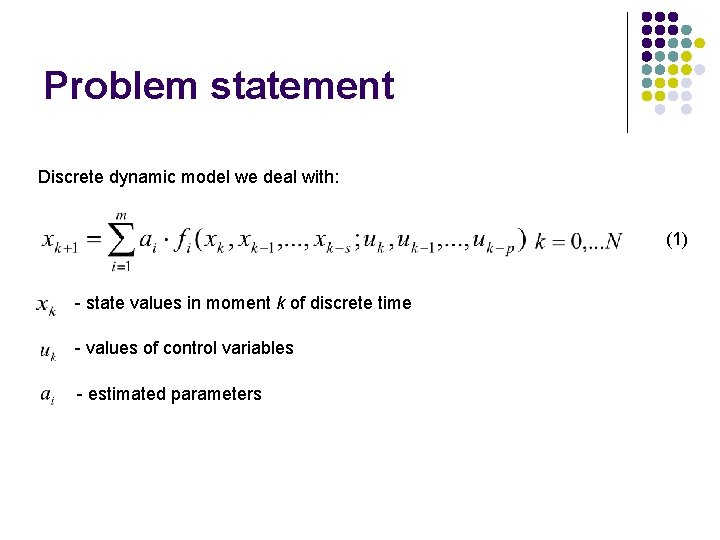Problem statement Discrete dynamic model we deal with: (1) - state values in moment k of discrete time - values of control variables - estimated parametersProblem statement We will denote matrix and vector - observed noise-corrupted state values - measured values of control variablesMethod of Least Squares estimations The difference that occur in model equations for observed state values leads to a system of normal Gaussian equations - vector of estimated model parametersUsage of equivalent integral form for dynamic equation Rewriting model equation (1) in difference form We can get an equivalent integral form of differential equations This leads to another system of normal Gaussian equations Where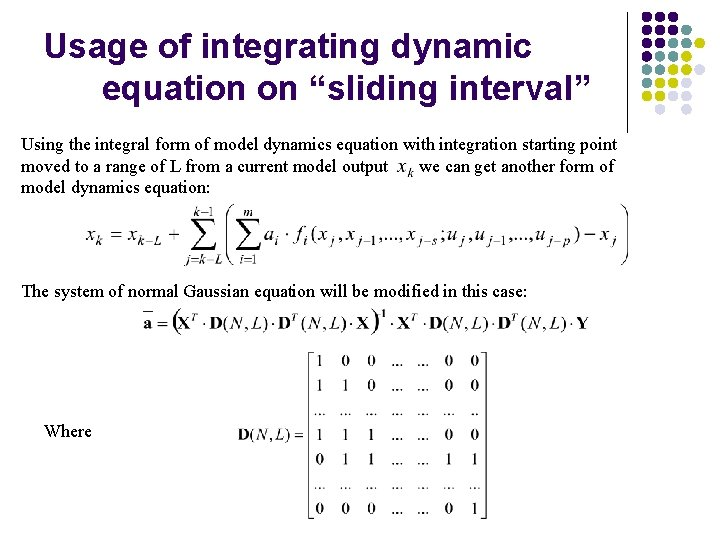Usage of integrating dynamic equation on “sliding interval” Using the integral form of model dynamics equation with integration starting point moved to a range of L from a current model output we can get another form of model dynamics equation: The system of normal Gaussian equation will be modified in this case: Where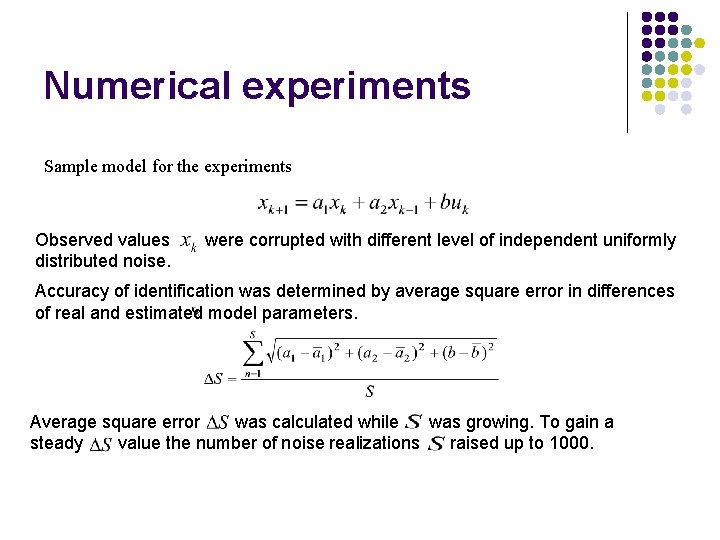Numerical experiments Sample model for the experiments Observed values distributed noise. were corrupted with different level of independent uniformly Accuracy of identification was determined by average square error in differences of real and estimated model parameters. Average square error was calculated while was growing. To gain a steady value the number of noise realizations raised up to 1000.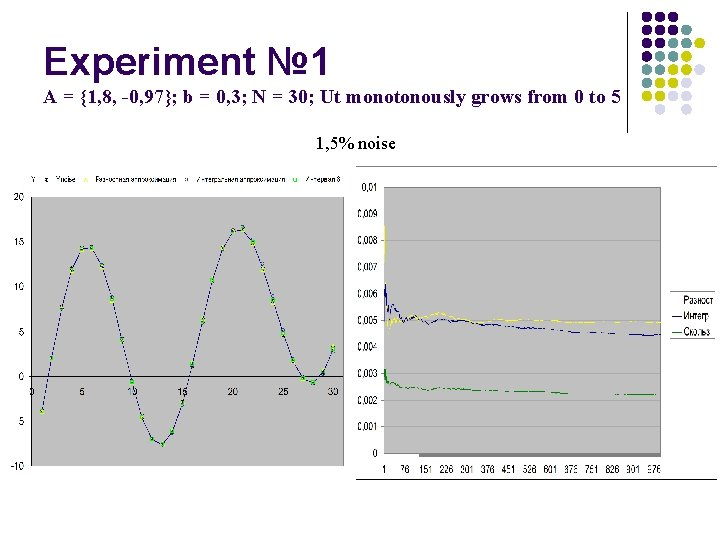Experiment № 1 A = {1, 8, -0, 97}; b = 0, 3; N = 30; Ut monotonously grows from 0 to 5 1, 5% noiseExperiment № 2 A = {1, 8, -0, 97}; b = 0, 3; N = 30; Ut monotonously grows from 0 to 5 5% noise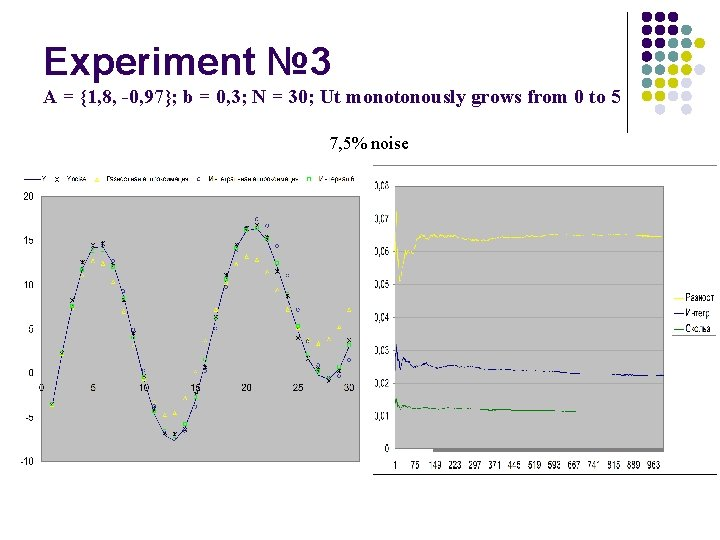Experiment № 3 A = {1, 8, -0, 97}; b = 0, 3; N = 30; Ut monotonously grows from 0 to 5 7, 5% noiseExperiment № 4 A = {1, 8, -0, 97}; b = 0, 3; N = 30; Ut monotonously grows from 0 to 5 15% noise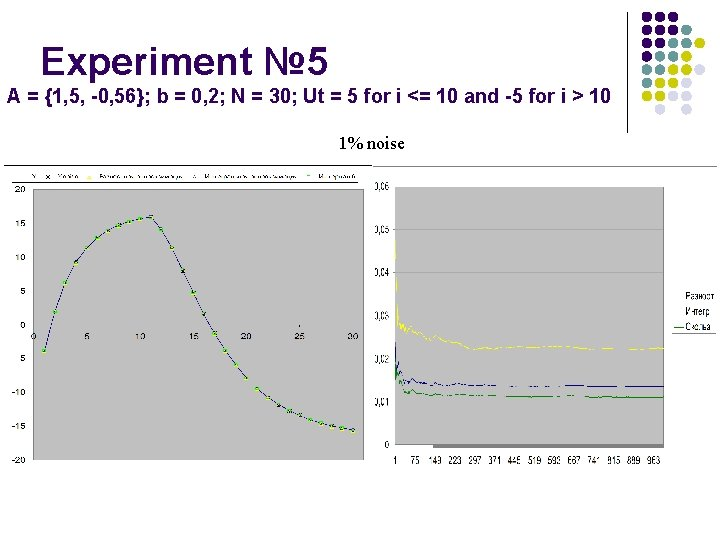Experiment № 5 A = {1, 5, -0, 56}; b = 0, 2; N = 30; Ut = 5 for i <= 10 and -5 for i > 10 1% noiseExperiment № 6 A = {1, 5, -0, 56}; b = 0, 2; N = 30; Ut = 5 for i <= 10 and -5 for i > 10 3% noise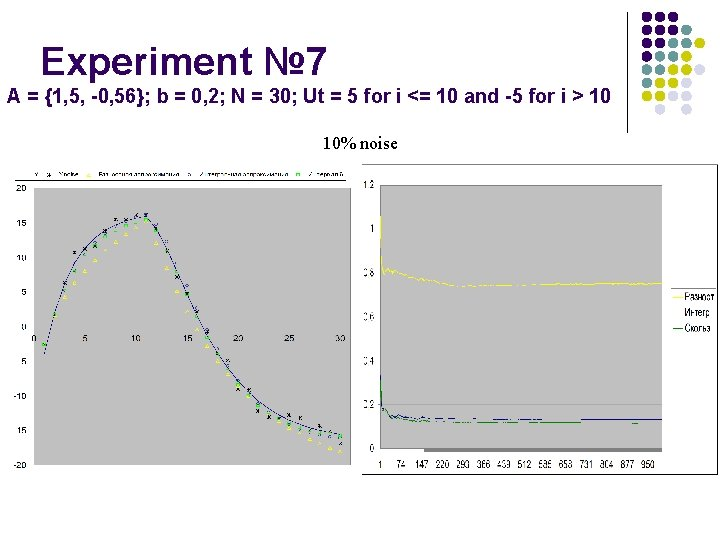Experiment № 7 A = {1, 5, -0, 56}; b = 0, 2; N = 30; Ut = 5 for i <= 10 and -5 for i > 10 10% noise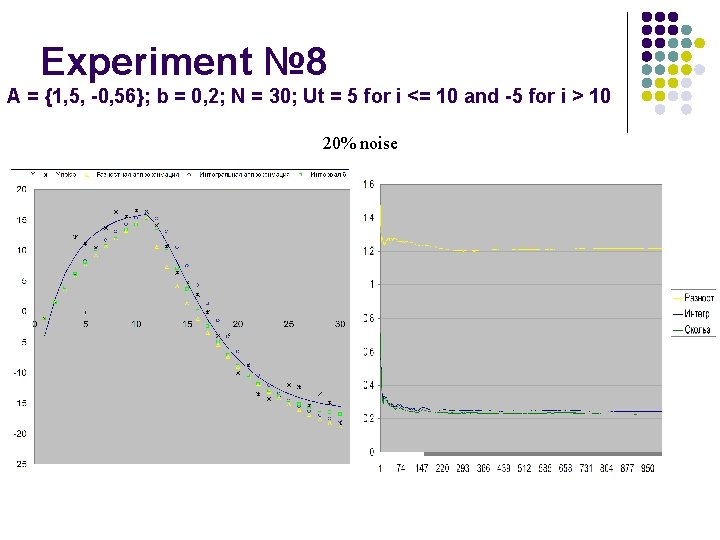Experiment № 8 A = {1, 5, -0, 56}; b = 0, 2; N = 30; Ut = 5 for i <= 10 and -5 for i > 10 20% noiseExperiment № 5 varying the length of “sliding interval”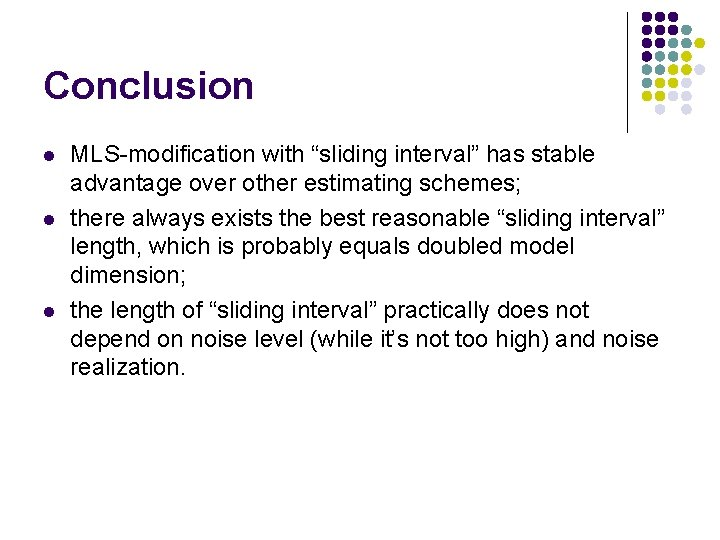Conclusion l l l MLS-modification with “sliding interval” has stable advantage over other estimating schemes; there always exists the best reasonable “sliding interval” length, which is probably equals doubled model dimension; the length of “sliding interval” practically does not depend on noise level (while it’s not too high) and noise realization.